# Differential scanning calorimeter facts for kids

Kids Encyclopedia Facts

Differential scanning calorimetry (DSC), is an analysis tool widely used in materials sciences, thermochemistry, drug purity and food quality testing. Its speed and ease of operation give instant information about the thermodynamic characteristics that play an important role in understanding complex processes during the formation of substances; for instance, polymer cross-linking, heat exchange due to folding and unfolding of proteins or formation mechanism of single or double stranded DNA. The DSC was firstly invented in 1962 by E.S. Watson and M.J. O’Neil and made available to the market in 1963 at the Analytical Chemistry and Applied Spectroscopy conference held in Pittsburgh.

## Physical Structure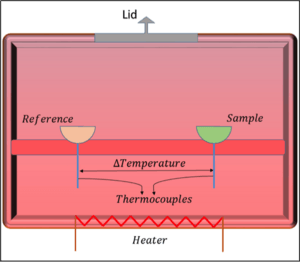Figure 1. Schematic drawing of heat flux Differential Scanning Calorimetry.

The two most common types of differential scanning calorimeter, are heat flux DSC, which operates by keeping the heat supply to the system as constant and power-compensated DSC, which works by maintaining the power supplied to the calorimeter as constant. In general, a DSC calculates heat changes by measuring the temperature difference between the sample and reference holder. The typical design of the heat flux DSC can be seen from Figure 1. It contains the sample holder, where the material of interest is placed, and the reference holder, which is generally kept empty. Both of these are placed on a support, which is in good contact with the walls of calorimeter. The heating resistor is attached to the boundary walls that enables one to obtain a furnace generating and keeping the required heat amount inside the enclosure. The thermocouple connected to both sample and reference holder is a measuring device that gives the temperature to be used in the analysis. Heat supplied by the heating resistor flows further into the sample and the reference materials chambers.

## Theory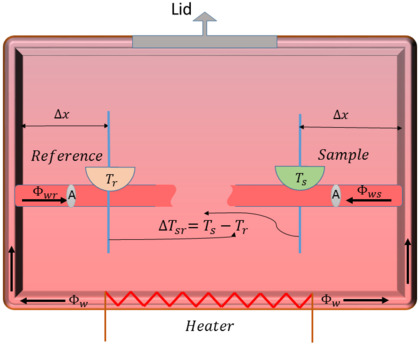Figure 2.The schematic drawing with all variables assigned for theoretical analysis.

The simplest theoretical approach to understand the working mechanism of DSC’s is called the simplified linear model and makes use of the following assumptions:

1. The heat flow rate is constant,
2. No interaction between the sample and reference,
3. Only heat capacities of sample and reference are considered,
4. The temperature being measured to be the current temperature of sample,
5. The system is isolated from surrounding, that is no heat exchange with outside.

The Fourier’s law of heat conduction, which is the fundamental law explaining how the heat is transferred through materials can be used to see the relationship between the temperature and the heat flow in the system. This law states that the amount of heat energy passing through a small portion of an area (A) of a material, which is called heat flux density and denoted by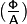${\textstyle (\frac{\mathsf{\Phi}}{\mathsf{A}}) }$ is equivalent to thermal conductivity (k) multiplied by the change in the temperature with respect to position, which can be denoted as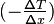${\textstyle (- \frac{\mathsf{\Delta}T}{\mathsf{\Delta}x}) }$. This relationship in an equation form can be written as,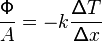$\quad\quad\quad\quad\quad\quad\quad\quad\quad\quad\quad\quad\quad\quad\quad\frac{\mathsf{\Phi}} {A} = -k \frac{\mathsf{\Delta} T} {\mathsf{\Delta} x}$

Generally, in DSC a computer is used to supply heat at specific rate into both the reference and sample holders. When the sample holder contains a substance while the reference kept empty leads to either increase or decrease in temperature of sample holder denoted by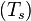${\textstyle (T_s)}$ depending on the following processes:

1. If the process is heat-consuming, that is external heat is needed for the event to take place, which is also called endothermic then the temperature in the sample holder decreases.
2. If the process is heat-releasing, that is extra heat is produced, which is also called exothermic then the temperature in the sample holder increases.

Then, the resulting change in the heat flow due to these temperature variations can be found by using the Fourier's law as the following,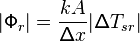$|\mathsf{\Phi}_r|=\frac {kA}{\mathsf{\Delta} x} |\mathsf{\Delta}T_{sr}|$ or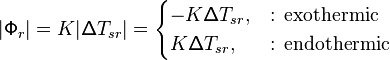$|\mathsf{\Phi}_r|=K|\mathsf{\Delta}T_{sr}|= \begin{cases} -K\mathsf{\Delta}T_{sr}, & \text{: exothermic} \\ K\mathsf{\Delta}T_{sr}, & \text{: endothermic} \end{cases}$

Hence, based on this simple model it turns out that there is a direct proportionality(K) between the heat flow and the temperature variations of the sample. This proportionality constant is dependent on the distance from the wall to the sample shown as (Δx), the cross section area of the support (A) and the thermal conductivity (k). Generally, the main results of the DSC experiment is an output signal of heat flow rate as a function of temperature, which are called DSC curves . Analysis of these curves plays an important role in determination of heat of transformation, heat of reactions or any changes in heat capacity due to temperature variations, for instance, the enthalpy of exothermic and endothermic processes can be determined by finding the area under the DSC curve using a mathematical technique called integral calculus.

## Applications

### Thermal denaturation of proteins

One of the most important applications of DCS is related to the thermal unfolding of the proteins, a process called denaturation.The DCS’s role in this process is used to determine the temperature range over which proteins exhibit structural changes. In addition to that, when the protein solution is treated under constant heat rate and constant pressure, DSC can determine apparent heat capacities of the proteins. In fact, the denatured proteins turn out to have higher heat capacities and proper detection of changes on them over time can help to find out the extent of unfolding.

### Evaluation of lipids and fats

Food quality control is one of the most important issues for human health care and well-being. There has been reported many unlawful practices with regard to food products, specifically adulteration of some highly priced vegetable oils and fats. Adulteration is an action of mixing low quality and sometimes harmful ingredients with food products intended to be sold. In this field, DSC is used to analyze the thermal behaviour of lipids mainly by two processes, the cooling process, which reports information about the crystallisation and the heating process, which gives information on the melting behaviour of the building blocks of lipids. The adulteration in fats or oils changes the DSC cooling and heating curves. For example, new peaks appear and existing peaks undergo changes. Hence, analysis of DSC data can be used in estimation of adulteration process on nutrients.

### Drug purity

DSC has gained quite a lot interest in drug purity investigation because it requires samples with low quantity (1–2 mg) and is considerably fast in terms of analysis time. For instance, by monitoring the effects of foreign substances, it can be found to what extent a drug is pure. It turns out that impurities decrease the melting temperature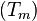$(T_m)$ of the drug. In addition, melting temperature can also be used to estimate the thermal stability of drugs, because the higher the$(T_m)$ the more stable is the protein. Therefore, DSC allows instant monitoring of this temperature leading to much easier and faster way of controlling the drug quality.

• Thomas Engel, Philip Reid, Physical Chemistry (3rd Edition), Prentice Hall (2013)
• Dr. G. W. H. Höhne, Dr. W. F. Hemminger, Dr. H.-J. Flammersheim, Differential Scanning Calorimetry. Springer (2003)
• J. L. McNaughton, C. T Mortimer, Differential Scanning Calorimetry. Perkin-Elmer Corp, CRC (1975). Edited by Emma Chiavaro.
• Julian M. Sturtevant, Biochemical Applications of Differential Scanning Calorimetry. Ann. Rev. Phys. Chem. 1987. 38: 463-88.
• Michael H. Chiu, Elmar J. Prenner, Differential scanning calorimetry: An invaluable tool for a detailed thermodynamic characterization of macromolecules and their interactions. J Pharm Bioallied Sci. 2011 Jan-Mar; 3(1): 39–59.
• Ilian Jelesarov, Hans Rudolf Bosshard, Isothermal titration calorimetry and differential scanning calorimetry as complementary tools to investigate the energetics of biomolecular recognition. J. Mol. Recognit. 1999;12:3–18
• McElhaney RN, The use of differential scanning calorimetry and differential thermal analysis in studies of model and biological membranes. Chem Phys Lipids. 1982;30:229–59.
• Bruylants G, Wouters J, Michaux C. Differential scanning calorimetry in life science: Thermodynamics, Stability, Molecular recognition and application in drug design.Curr Med Chem. 2005;12:2011–20.

## Images for kidsDifferential scanning calorimeter Facts for Kids. Kiddle Encyclopedia.